##### Geometry: 1,001 Practice Problems For Dummies (+ Free Online Practice)You can apply your knowledge of geometric constructions to solve a variety of problems. The following practice questions test your ability to construct parallel and perpendicular lines.

If you're drawing two arcs for a construction, make sure you keep the width of the compass (or radii of the circles) consistent, and make your arcs large enough so that they intersect.

## Practice questions

1. Use the following diagram to construct a line perpendicular to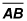through Point C.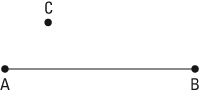2. Construct a line parallel to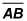that passes through Point C.1. Here is the solution: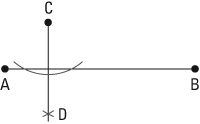Place the compass point on C, and using any width, draw an arc that intersects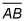twice.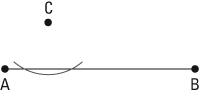Place the compass point at both locations where the arcs intersect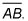At each location, make an arc belowLabel the intersection of those arcs D and connect D to C.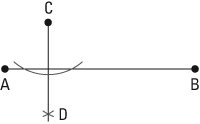2. Here is the solution: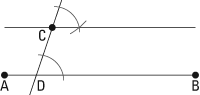Draw a line from Point C throughat an angle. Label the intersection Point D.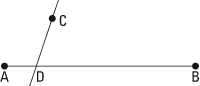Using a width about half of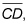place the compass point at D and draw one large arc. Using the same compass width, do the same at Point C.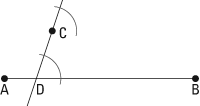Measure the width of the first arc. Using that same width, place the compass point at C and draw an arc intersecting the original arc you drew.

Connect C to the point where the arcs intersect.